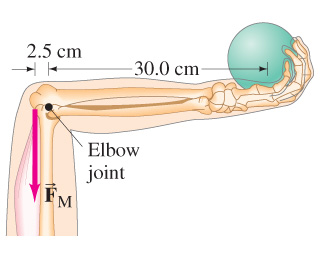# Approximately what magnitude force, FM, must the extensor muscle in the upper arm exert on the...

## Question:

Approximately what magnitude force, FM, must the extensor muscle in the upper arm exert on the lower arm to hold a 4.1-kg shot put?

Assume the lower arm has a mass of 2.9 kg and its CG is 13 cm from the elbow-joint pivot.

## Torque:

When an object rotates about an axis. it experiences a torque. One example of torque is the door that rotates about its joint to the wall. Torque is equal to the cross product of force and the perpendicular distance from the axis of rotation.

{eq}\vec \tau = \vec r \times \vec F {/eq}Torque is given by,

{eq}\tau = r \times F {/eq}

Where, {eq}F {/eq} is the force and {eq}r {/eq} is the perpendicular distance from the axis of the rotation.

From the extensor muscle force ({eq}F_M {/eq}, the elbow-joint pivot is {eq}\rm 2.5 \times 10^{-2} \ m {/eq} away. The origin is assume to be at joint.

Torque at Elbow-Joint Pivot is,

{eq}\tau_1 = \rm F_M *(-2.5) * 10^{-2} {/eq}

The lower arm is {eq}\rm 13 \times 10^{-2} \ m {/eq} away from the elbow-joint pivot. Torque experienced by the lower arm from the elbow-joint pivot point is,

{eq}\tau_2 = m*CG*g = \rm 2.9 *13*10^{-2}*9.8 {/eq}

Where, {eq}m {/eq} is the mass of the lower arm. {eq}CG {/eq} is the center of gravity. {eq}g {/eq} is the acceleration due to gravity.

The {eq}\rm 4.1 \ kg {/eq} shot put is held by the upper arm is at {eq}\rm 30 \times 10^{-2 } \ m {/eq} away from the elbow-joint pivot.

{eq}\tau_3 = \rm 4.1 * 9.8 * 30*10^{-2 } {/eq}

Then,

{eq}\rm - F_M *2.5 * 10^{-2} + 2.9 *13*10^{-2}*9.8 + 4.1 * 9.8 * 30*10^{-2 } = 0 {/eq}

{eq}\rm F_M = \rm 630 \ N {/eq}